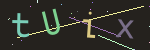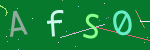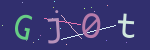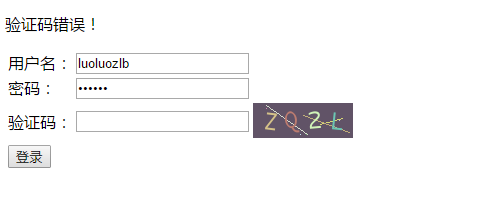# 什么是GD库

PHP手册中的介绍：

PHP 并不仅限于创建 HTML 输出， 它也可以创建和处理包括 GIF， PNG， JPEG， WBMP 以及 XPM 在内的多种格式的图像。 更加方便的是，PHP 可以直接将图像数据流输出到浏览器。 要想在 PHP 中使用图像处理功能，你需要连带 GD 库一起来编译 PHP。 GD 库和 PHP 可能需要其他的库， 这取决于你要处理的图像格式。

# GD库的使用方法

GD库是用来生成和处理图像的，使用GD库处理图像分为四个步骤：
1. 创建画布：画布的类似于我们在画画时使用的画布，画布可以是新创建的或者从图像文件读取的。
2. 处理图像：创建画布成功以后，就使用各种GD库函数处理图像了，可以设置图像的颜色、填充画布、画点、线段、各种几何图形，以及向图像中添加文本等。
3. 输出图像：处理完成后，可以将图片发送到浏览器或者保存到文件中。
4. 释放资源：这一步可以省略，因为php脚本结束后会自动释放资源

# 使用GD库生成图像验证码

## 生成图像验证码的步骤

1. 创建新画布并填充背景色
2. 添加图像中的干扰项，这些干扰项可以是圆弧、线条、点等
3. 添加验证码内容, 这些内容一般是随机生成的四个数字或者字母
5. 释放资源，这步可以省略

## 代码例子

（大家可以拿去直接使用）

<?php
/**
* 生成图像验证码
* 可以通过GET方法传入width和height设置图片大小
* 生成之后通过$_SESSION["vcode"]获取验证码 * @author luoluozlb <643552878@qq.com> 2017/6/15 */$width = 150;
$height = 50; if(isset($_GET['width'])){
$width =$_GET['width'];
}
if(isset($_GET['height'])){$height = $_GET['height']; }$fontSize = $height / 2;$fontFile = 'Monaco.ttf';  //字体文件位置

//随机产生一个背景颜色(暗色)
function RandomBackColor($imgSource){ return imagecolorallocate($imgSource, mt_rand(0, 128), mt_rand(0, 128),  mt_rand(0, 128));
}

//随机产生一个颜色(亮色)
function RandomColor($imgSource){ return imagecolorallocate($imgSource, mt_rand(100, 255), mt_rand(100, 255),  mt_rand(100, 255));
}

$img = imagecreatetruecolor($width, $height); //创建画布 imagefill($img, 0, 0, RandomBackColor($img)); //填充背景 //添加一些干扰直线 for($i = 0; $i < 3; ++$i){
imageline($img, mt_rand(0,$width), mt_rand(0, $height), mt_rand(0,$width), mt_rand(0, $height), RandomColor($img));
}

//添加一些干扰弧线
for($i = 0;$i < 3; ++ $i){ imagearc($img, mt_rand(- $width,$width), mt_rand(- $height,$height), mt_rand(0, $width), mt_rand(0,$height), mt_rand(0, 360), mt_rand(0, 360), RandomColor($img)); } //添加一些干扰点 for($i = 0; $i < 25; ++$i){
imagesetpixel($img, mt_rand(0,150), mt_rand(0,60), RandomColor($img));
}

//生成验证码
$codeRange = '0123456789abcdefghijkmnpqrstuvwxyzABCDEFGHIJKLMNPQRSTUVWXYZ'; //验证码字符的取值范围$code = '';
for($i = 0,$len = strlen($codeRange);$i < 4; ++ $i){ //循环4次，就是有四个随机的字母或者数字$code .= $codeRange[mt_rand(0,$len - 1)];
}

//添加验证码到图像
$x = 10;$dx = ($width - 10)/4;$y = $height - ($height - $fontSize)/2; for($i = 0; $i < 4; ++$i){
imagettftext($img,$fontSize, mt_rand(-15, 15), $x,$y, RandomColor($img),$fontFile, $code[$i]);
$x +=$dx;
}

session_start();
$_SESSION["vcode"] =$code;    //验证码保存到seesion中
imagepng($img); // 输出图像 imagedestroy($img);         // 销毁图像
?>## 在登录页面中使用图像验证码

<?php
$user =$password = $errmsg = ''; if($_SERVER['REQUEST_METHOD'] == 'POST'){
$user =$_POST['user'];
$password =$_POST['password'];
//验证用户名和密码
//code...

//验证验证码
session_start();
if(empty($_POST['vcode'])){$errmsg = "请填写验证码！";
}
else{
if(0 == strcasecmp($_POST['vcode'],$_SESSION['vcode'])){  //不区分大小写比较
echo '登录成功！';
exit();
}else{
$errmsg = "验证码错误！"; } } } ?> <!DOCTYPE html> <html> <head> <meta charset="UTF-8" /> <title>用户登录</title> </head> <body> <p><?php echo$errmsg; ?></p>
<table>
<tr>
<td><label for='user'>用户名：</label></td>
<td><input type='text' name='user' id='user' value='<?php echo $user; ?>' /></td> </tr> <tr> <td><label for='password'>密码：</label></td> <td><input type='password' name='password' id='password' value='<?php echo$password; ?>' /></td>
</tr>
<tr>
<td><label for='vcode'>验证码：</label></td>
<td><img src="vcode.php?width=100&height=35" alt="验证码"></td>
</tr>
<tr>
<td><input type="submit" value='登录'></td>
</tr>
</table>
</form>
</body>
</html>## 完整的源码下载

07-164092

#### PHP 使用GD库生成验证码 在图像上绘制汉字12-291966

#### PHP生成图片验证码

01-031611

#### php使用GD库生成验证码

10-26574

#### 利用PHP应用图像处理生成验证码

01-1221

#### PHP5中GD库生成图形验证码

01-07204

#### PHP使用GD函数库创建验证码+调用

07-15404

#### 应用PHP GD库中图像处理函数制作验证码

09-01370

#### php制作验证码用到的函数

10-123631

#### php通过GD库实现验证码

06-161985

#### PHP图片水印类（GD库）

12-30190

#### php生成各种验证码

12-0618

#### PHP验证码生成及图片处理（GD库）

05-11132

#### PHP生成验证码

07-26542

#### php生成图片验证码，使用javascript点击图片刷新验证码，以及后台验证验证码是否正确

01-0466

#### GD库生成图片验证码

09-12266

#### GD库 验证码函数

05-05119

#### GD库问题验证码不显示分析处理

04-16338

#### PHP5验证码无法显示，GD库正常安装也无法显示的解决方法©️2020 CSDN 皮肤主题: 大白 设计师: CSDN官方博客点击重新获取扫码支付1.余额是钱包充值的虚拟货币，按照1:1的比例进行支付金额的抵扣。
2.余额无法直接购买下载，可以购买VIP、C币套餐、付费专栏及课程。余额充值